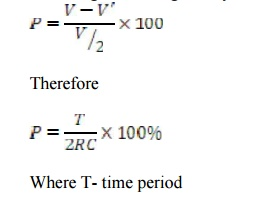Home | | Electronic Circuits II | Important Short Questions and Answers: Wave Shaping and Multivibrator Circuits

# Important Short Questions and Answers: Wave Shaping and Multivibrator Circuits

Electronic Circuits - Wave Shaping and Multivibrator Circuits - Important Short Questions and Answers: Wave Shaping and Multivibrator Circuits

WAVE SHAPING CICUITS AND MULTIVIBRATORS

1.    Give two applications of bistable multivibrators

·              Used to generate symmetrical square wave. This is possible by using triggering pulses of equal interval, corresponding to the frequency required.

·              Used as a memory element in shift registers,counters,etc,.

·              Used for the performance of many digital operations like counting and storing of digital information.

·              Can be used as a frequency divider

2.    How does a diode act as a comparator?

A Comparator Circuit is used to identify the instant at which the arbitrary input waveform attains a particular reference level. Basically it is a clipper circuit. A simple diode comparator and its equivalent circuit is given as3.    What is meant by clipper circuit?

The electronic circuits which are used to clip off the unwanted portion of the waveform, without distorting the remaining part of the waveform are called clipper circuits.

4.    What is the 'tilt' applicable to RC circuits? Give an expression for tilt.

In high pass RC network, Tilt is defined as the decay in the amplitude of the output voltage waveform, when the input maintains its level constant.

Percentage of tilt is given by5.    What type of distortion is observed in astable multivibrator?

It can be seen that, in the collector waveforms shown in the figure there is certain distortion present. Instead of [Vc1, Vc2] exact square wave, we are getting the vertical rising edges little bit rounded. This is called rounding. For a square wave output such a rounding is undesirable and must be eliminated.

6.    What is meant by clamper circuit?

The electronic circuits which are used to add a dc level as per the requirement to the ac output signal are called clamper circuits.

It is also known as dc inserter or dc restorer.

7.           Give two applications of Schmitt Trigger circuit..

· It is used as a amplitude comparator

· It can be used as a squaring circuit.

·   It can be used as a sine wave to square wave converter

8.           Why do we call astable multivibrator as free running multivibrator?

An astable multivibrator is called free running multivibrator because it generates square waves of its own without any external triggering pulse.

9.    Define the threshold points in a Schmitt trigger circuit.

·              Schmitt trigger is a type of comparator with two different threshold voltage levels on points (UTP, LTP).

·              Whenever the input signal goes over the high threshold levels, the output of the comparator is switched high. The output will remain in this same state as long as the input voltage is above the low threshold level.

·              When the input voltage goes below this level, the output will switch. These threshold voltage levels are called threshold points.

10. What is a regenerative comparator? Give example circuit.

Regenerative comparator is a circuit, compares its input voltage to a “threshold voltage”, because it has two threshold voltages(the upper and lower trigger voltages). The threshold voltage depends on the output state. If the input voltage is higher than the upper trigger voltage, the output will be high.

A small amount of the output voltage is effectively added to the input voltage before it is compared to a fixed threshold. So it uses positive (or) regenerative feedback.

E.g.: Schmitt Trigger

11. Distinguish between symmetrical and unsymmetrical triggering methods.

Symmetrical triggering uses only one trigger input and unsymmetrical triggering uses two trigger input.

12.      Why Monostable multivibrator is also called as delay circuit?

Used to introduce time delay as gate width is adjustable.

13.      Determine the value of capacitors to be used in an astable multivibrator to provide a train of pulse of 4 μs wide at a repetition rate okf 80KHz if R1=R2=10K.

14.      A 20 KHz, 75% duty cycle square wave is used to trigger continuously, a monostable multivibrator with triggered pulse duration of 5µs. What will be the duty cycle of the output of the monostable multivibrator?

15.      A RC low pass circuit has R=1.5Kohm and C=0.2micro farad. What is the rise time of the output when excited by a step input.

16.      In a low pass RC circuit, rise time is 35 nano seconds. What is the bandwidth that can be obtained using the circuit?

17.      Determine the value of capacitors to be used in an astable multivibrator to provide a train of pulse 2µs wide at a repetition rate of 75KHz with R1=R2=10KHz.

Study Material, Lecturing Notes, Assignment, Reference, Wiki description explanation, brief detail
Electronic Circuits : Wave Shaping and Multivibrator Circuits : Important Short Questions and Answers: Wave Shaping and Multivibrator Circuits |